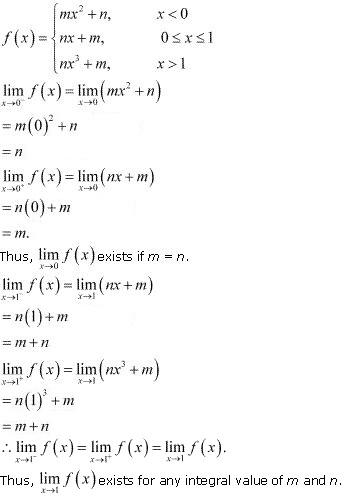# Class 11 Maths NCERT Solutions for Chapter 13 Limits and Derivatives Exercise 13.1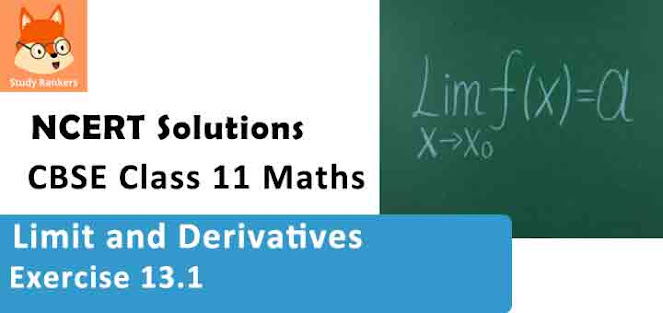### Limits and Derivatives Exercise 13.1 Solutions

1. Evaluate the Given limit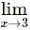(x + 3)

Solution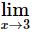(x + 3) = 3 + 3 = 6

2. Evaluate the Given limit :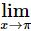(x - 22/7)

Solution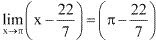3. Evaluate the Given limit :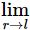πr2.
Solutionπr2 = π(1)2 = π

4. Evaluate the Given limit :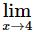(4x + 3)/(x - 2)
Solution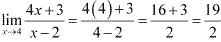5. Evaluate the Given limit :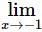(x10 + x5 + 1)/(x – 1)
Solution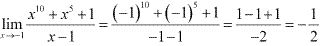6. Evaluate the Given limit :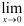[(x + 1)5 - 1]/x
Solution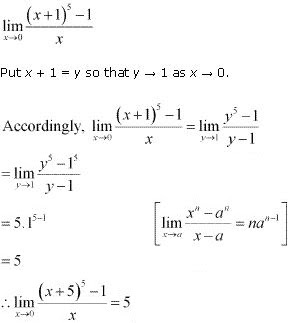7. Evaluate the Given limit :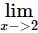(3x2 - x - 10)/(x2 - 4)
Solution
At x = 2, the value of the given rational function takes the form 0/0 .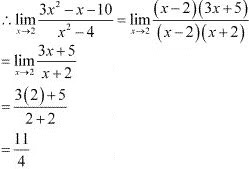8. Evaluate the Given limit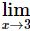(x4 -81)/(2x2 - 5x - 3)
Solution
At x = 2, the value of the given rational function takes the form 0/0 .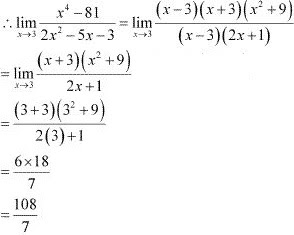9. Evaluate the Given limit :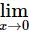(ax + b)/(cx + 1)
Solution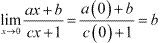10. Evaluate the Given limit :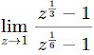Solution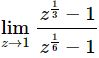At z = 1, the value of the given function takes the form 0/0 .
Put z1/6 = x so that z →1 as x →1.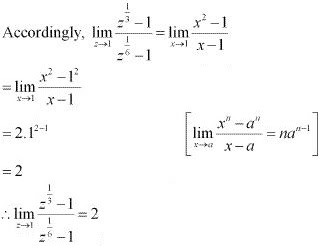11. Evaluate the Given limit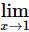(ax2 + bx + c)/(cx2 + bx + a) , a + b + c ≠ 0 .
Solution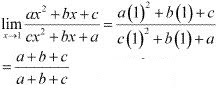= 1 [a + b + c ≠ 0 ]

12. Evaluate the Given limit :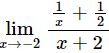SolutionAt x = -2, the value of the given function takes the form  0/0 .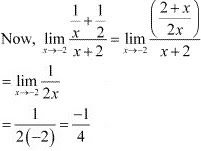13. Evaluate the Given limitsin ax/bx
Solutionsin ax/bx
At x = 0, the value of the given function takes the form 0/0 .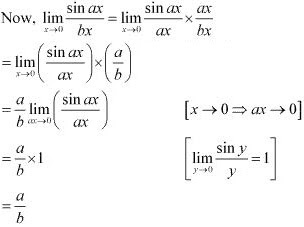14. Evaluate the Given limit :sin ax/sin bx, a,b ≠ 0

Solution(sin ax/sin bx), a,b ≠ 0
At x = 0, the value of the given function takes the form  0/0.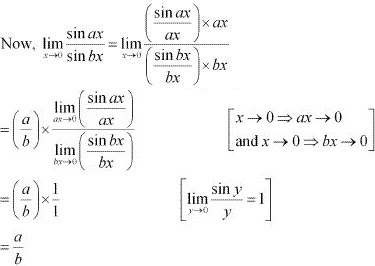15. Evaluate the Given limit[sin(π -x)]/π(π - x)
Solution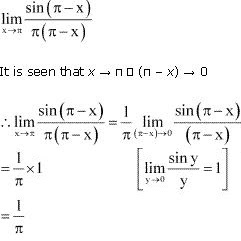16. Evaluate the given limitcos x/(π - x)
Solution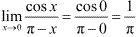17. Evaluate the Given limit :(cos 2x - 1)/(cos x- 1)
Solution(cos 2x - 1)/(cos x- 1)
At x = 0, the value of the given function takes the form  0/0 .
Now,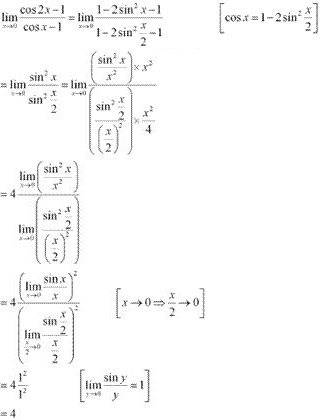18. Evaluate the Given limit(ax + x cos x)/b sin x
Solution(ax + x cos x)/b sin x
At x = 0, the value of the given function takes the form 0/0.
Now,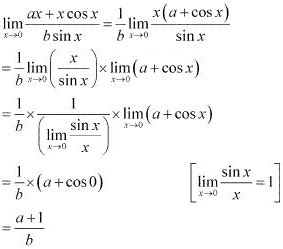19. Evaluate the Given limitsec x
Solution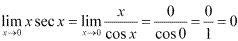20. Evaluate the Given limit(sin ax + bx)/(ax + sin  bx) a,b, a+b ≠ 0.
Solution
At x = 0, the value of the given function takes the form 0/0 .
Now,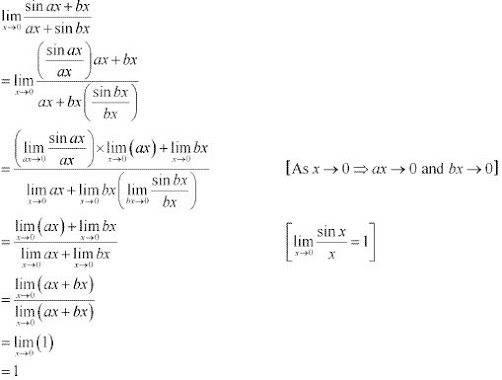21. Evaluate the Given limit(cosec x - cot x)
Solution
At x = 0, the value of the given function takes the form ∞→∞.
Now,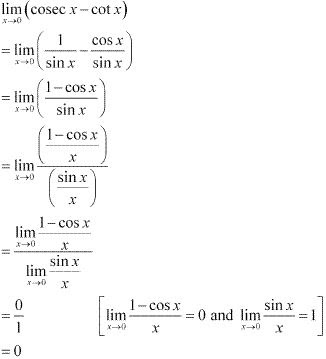22. Evaluate the Given limit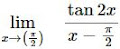Solution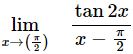At x = π/2 , the value of the given function takes the form  0/0.
Now, put x - π/2 = y so that x → π/2, y → 0.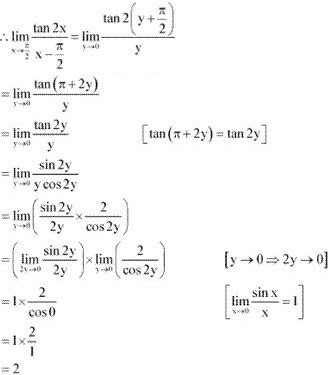23. Findf(x)  andf(x) where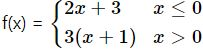Solution
The given function is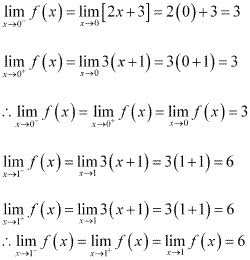24. Findf(x) where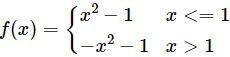Solution
The given function is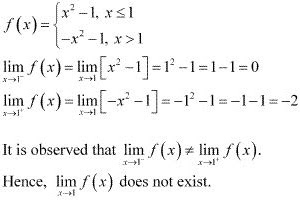25. Evaluatef(x) where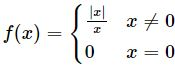Solution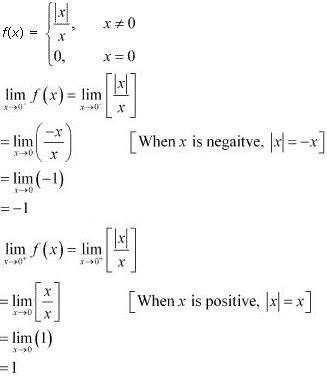It is observed thatf(x) ≠f(x).
Hence,f(x) does not exist.

26. Findf(x) where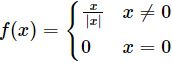Solution
The given function is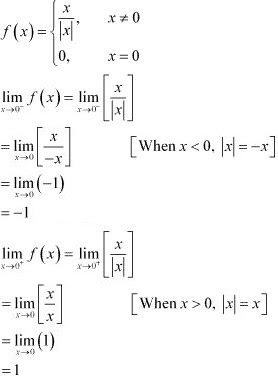It is observed thatf(x) ≠f(x).
Hence,f(x) does not exist .

27. Find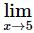f(x) , where f(x) = |x| - 5
Solution
The given functions is f(x) = |x| - 5.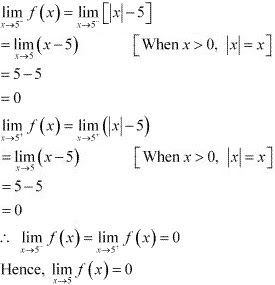28. Suppose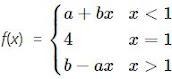and iff(x) = f(1) what are possible values of a and b?
Solution
The given function is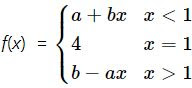f(x) =(a + bx) = a + bf(x) =(b - ax) = b - a
f(1) = 4
It is given thatf(x) = f(1).
∴f(x) =f(x) =f(x) = f(1)
⇒ a + b = 4 and b - a = 4
On solving these two equations, we obtain a = 0 and b = 4.
Thus, the respective possible values of a and b are 0 and 4.

29. Let a1, a2, . . ., an be fixed real numbers and define a function f ( x) = (x − a1) (x − a2)...(x − an). What is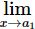f(x) ? For some a ≠ a1, a2, ..., an, compute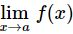Solution
The given function is  f(x) = (x – a1)(x – a2) … (x - an).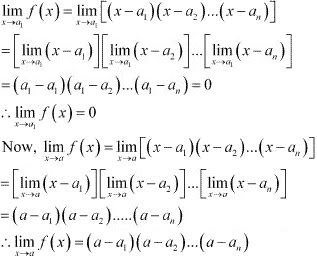30. If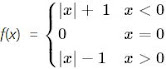For what value (s)  of a does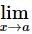f(x) exists ?
Solution
The given function is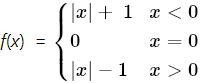When a = 0,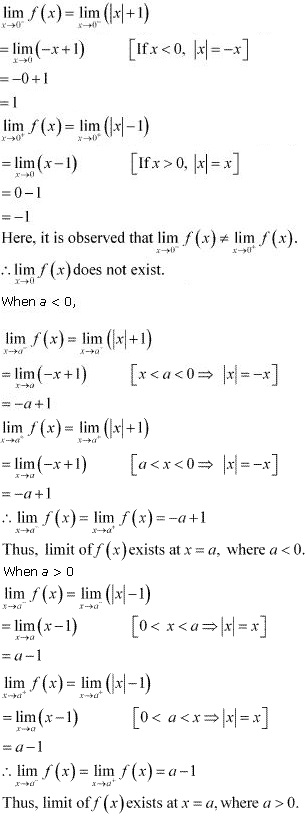31. If the function f(x) satisfies[f(x) - 2]/[x2 -1] = π , evaluatef(x).
Solution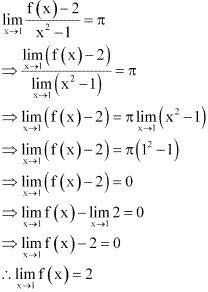32. If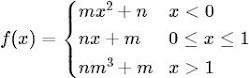For what integers m and n doesf(x) andf(x) exist ?
Solution
The given function is